# Perimeter Calculator

Use this perimeter calculator to easily calculate the perimeter of common bodies like a square, rectangle, triangle, circle, parallelogram, trapezoid, ellipse, regular octagon, and sector of a circle. Formulas and explanation below.

Share calculator:

Embed this tool:
get code

## How to calculate the perimeter of any shape?

There are different rules for calculating the perimeter of different geometrical shapes. Our perimeter calculator supports a lot of the basic shapes and below you can read details about each one, including its perimeter calculation formula. When doing the calculation, remember to take each measurement in the same unit, or to convert it to the same unit, to get valid results.

## Perimeter of a square

The formula for the perimeter of a square is side x 4, as seen in the figure below: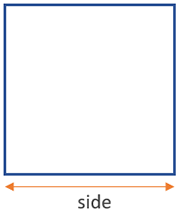This is the easiest shape to calculate, as you only need to take a single measurement. You barely even need a calculator for that. It is, however, also rarely encountered in practical questions.

## Perimeter of a rectangle

The formula for the perimeter of a rectangle is (width + height) x 2, as seen in the figure below: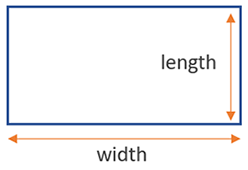You need two measurements for a rectangle - width and length. Make sure both are in the same unit, or convert one of them as necessary. Due to the simplicity of the shape, measurements are easy to take and a perimeter calculator simplifies the calculation only when the numbers are big.

## Perimeter of a triangle

The formula for the perimeter of a triangle is side a + side b + side c, but there are many rules through which one can calculate it. Visual in the figure below: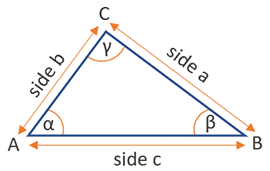Our perimeter calculator also supports the following rules: SAS (side, angle, side), SSA (side, side, angle), ASA (angle, side, angle) and the hypotenuse and side rule for right-angled triangles.

## Circumference of a circle

The formula for the circumference of a circle is 2 x π x radius, but the diameter of the circle is d = 2 x r, so another way to write it is 2 x π x (diameter / 2). Visual on the figure below: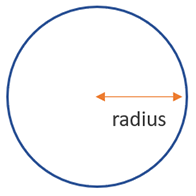In many practical situations it is easier to measure the diameter accurately, rather than the radius. Also, in many engineering schematics it is the circle diameter which is given by default, not the radius.

## Perimeter of a parallelogram

The formula for the perimeter of a parallelogram is (width + height) x 2, as seen in the figure below: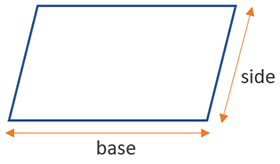A parallelogram's perimeter is calculated using the same formula as a rectangle, since in both shapes the opposite sides are equal in length.

## Perimeter of a trapezoid

The formula for the perimeter of a trapezoid is base 1 + base 2 + side a + side b, as seen in the figure below: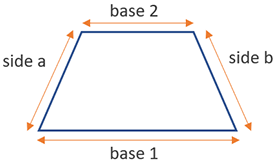You need more measurements for a trapezoid, as it is a more complex form in which all sides can have a different length.

## Circumference of an ellipse (oval)

There is no single formula for the circumference of an ellipse, as it is surprisingly difficult to calculate it accurately. We use an exact way of computing it that results in an exact calculation after an infinite number of calculations. If the number of calculations is less than infinite, there is a small error. It converges quickly to the true value, so we do several steps only.

First, the calculator calculates h = (major radius - minor radius)2 / (major radius + minor radius)2 . Then it computes the perimeter as equal to π x (major radius + minor radius) x (1 + h * 0.25 + h2 * (1/64) + h3 * (1/256) + h4 * (25/16384) + h5 * (49/65536) + h6 * (441/1048576). Visual on the figure below: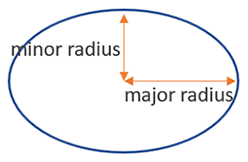## Perimeter of a sector

The formula for the perimeter of a sector is 2 x radius + radius x angle x (π / 360). Visual on the figure below: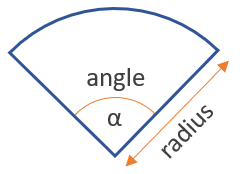A sector is just a part of a circle, so the formula is similar. The added complexity comes from the need to calculate how much of a circle a sector accounts for.

## Perimeter of an octagon

The formula for the perimeter of a regular octagon is side x 8, as shown in the figure below: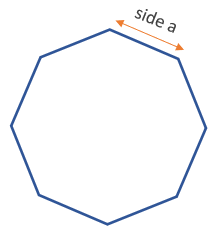This is one of the easiest shapes to calculate the perimeter of - only a single measurement is required, and a simple multiplication by eight is all the calculation that needs to be done. Regular octagons can be encountered in engineering, landscaping and gardening, and architecture.

## Where is a perimeter calculator useful?

Besides the obvious - tasks in geometry class or homework, a perimeter calculator can have many practical applications. For example, in sports - you may decide that you need to walk or run 10km per day to stay in good physical shape. However, what to do if there are no good tracks near you? You can simply choose a large building or any rectangular street block or set of blocks, calculate their perimeter and then divide 10km by it to determine how many laps you need to make.

A calculator can also be useful in a variety of DIY projects at home or in the garden, including in things like home decoration, needlework, and so on. Working in engineering and some crafts often ends up requiring some perimeter calculations.

#### Cite this calculator & page

If you'd like to cite this online calculator resource and information as provided on the page, you can use the following citation:
Georgiev G.Z., "Perimeter Calculator", [online] Available at: https://www.gigacalculator.com/calculators/perimeter-calculator.php URL [Accessed Date: 06 Jun, 2023].